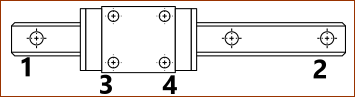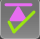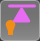﻿ Getting Started Tutorials - MechDesigner > Tutorial 13: Forces: Introduction  > Step 13.1B: Slider

# Step 13.1B: Slider

### SLIDER FORCES

This topic shows how Force-Vectors are calculated and displayed for a Slider.

Slider = Part + Slide-Joint + Motion-Dimension FB.

Note:

Motions for this Step: Motions for Tutorial13-1B  Download the ZIP file, extract the MTD.

Use MotionDesigner > Open and Append to load the motions into MotionDesigner.

#### The SliderThe image shows the Sliding-Part in the Part-Editor. The CAD-Line is at the top. The X-axis (in orange) is on top of the CAD-Line! A Line •40mm long is parallel to the CAD-Line •50mm below the CAD-Line In the next image, the kinematic-chain is a Slider - To help you see the dimensions and Point numbers in the image, I am not showing the Motion-Dimension - As is the definition, a Slide-Joint is constructed with 2 Lines; a Line in two different Parts. Each Line is defined by its start-Point and its end-Point - 4 Points in total. In the image, the numbers (1,2,3,4) identify the 4 Points: •Line 1 (defined by start-Point 1 and end-Point 2) of the Slide-Joint, in the Base-Part: Its length is 100mm. This Line is horizontal. It supports the sliding-Part. •Line 2 (defined by start-Point 3 and end-Point 4) of the Slide-Joint, in the Sliding-Part: Its length is 40mm(20+20). The Line is 50mm below the Part and CAD-Line. The Mass in the Sliding-Part is 1kg. The center-of-Mass is 50mm along the CAD-Line. We can add the mass and position the mass with the CAD-Line dialog-box | Mass Properties tab > User Mass Propertie In this example, the Mass is an idealized Point-Mass - the mass does not have an Inertia or a Radius-of-Gyration.Schematic- Linear Slide-Block and Slide-Rail. Approximate position of Reaction Points Notes: This is a schematic of a typical Linear Slider Rail with a Slide Block, that you might use in a machine design. 1, 2, 3, and 4 - in the image - are approximately equivalent to the start-Points and end-Points of the two Lines in a Slide-Joint In your model you should make sure that the Points (1,2,3,4) of the Lines in the Slide-Joint have the length equal to the length of the Slide-Rail and Sliding-Block.

#### Calculate and Display Forces1.Click Force toolbar > Calculate Forces so that the icon becomes the same as the icon to the left.2.Click Force toolbar > Display Force Vectors so that the icon becomes the same as the icon to the left If a minimum of one Part has Mass, MechDesigner displays Force Vectors. The Force Vectors show the direction, magnitude, and location of each Force. Note: you may need to click the Force and Torque Vector Scale to increase or decrease the length of the Force Vectors. These buttons are below the graphic area, in the middle of the Feedback Area. If necessary, change the background color of the graphic area to a Dark-Grey so that you can see the magnitude of each vector next to the arrowhead of each Force Vector. •There is a Force Vector from the start-Point and end-Point of each Line that defines the Slide-Joint - four(4) Points , and four(4) Force-Vectors •The four Points that make up a Slide-Joint can ONLY exert a force that is perpendicular(⊥) to o each Line. •If the sliding-part is a Slider, and it is a Power Source, then there is a Motive Force-Vector in the direction of the Slider.

#### CASE 1: Stationary or Constant Velocity SliderWhen the Sliding-Part moves along the Slide-Joint at Constant-Velocity, the inertia-forces are zero. Also, as the Sliding-Part is not rotating, the Coriolis-Force or Centrifugal Force are zero. Consider the Forces acting on the Sliding-Part. •Gravitational-Force: The Slider has a Mass of 1kg. Thus, there is a Gravitational Force = 9.81N. This force vector is not shown. •Inertia-Force: It is stationary, or moving at a constant-velocity. Thus, the Inertia Force = 0N The Gravitational Force must be put into equilibrium by reaction forces that act on the sliding-part. In the image, there are two, force-vectors, upwards, each equal to 4.90N,that ACT ON the sliding-part, by the Base-Part at Points 3 and Point 4. These two Force Vectors are equal because of the mass is above the mid-Point of the sliding-part, and the Slide-Joint is horizontal. Consider the Forces acting on the Base-Part. Refer to the dimensions and point numbers in the image at the top of this topic. The total force acting upwards by the Base-Part on the sliding-Part is 9.81N. •Moments, about Point 1: 0.020(m)*4.9(N) + 0.060(m)*4.9(N) - 0.100(m)*R4(N) = 0 ; R4 = 3.92N. •Vertical Forces: ∑FV: R1 + R2 + R3 + R4 = 0 ;  R1 = 5.88N These Force Vectors ACT ON the Base-Part, by the Sliding-Part.Note: Motion name for this video is CV.MTD   The Lines in the Slide-Joint should be thought of as a Linear Slide Rail and a Linear Slide Block, as you would purchase from THK. (other well know suppliers of Slide-Rails and Sliding-Blocks.   The Force Vectors on the Base-Part as the sliding-Part moves along it with Constant-Velocity. In reality, the forces would be distributed along the Lines in the two Parts. However, in MechDesigner, the Forces Vectors are at the Points at each end of each Line in the Slide-Joint.

#### Case 2: Forces on Accelerating Slider.Note: Download the motions (see top of this topic), set the Edit menu > Machine Settings > Cycling Parameters > Cycles/Min to 300. Add a Motion FB for the Slider and link ConstAcc to the Motion FB The acceleration of the sliding-part to the Right is 10m/s/s (Taking Acceleration to the Right (→) as Positive) Consider the Sliding-Part. •Gravitational Force, vertical ( | ) : 1kg*9.81m/s/s = 9.81N . Thus there is a gravitational force vertically downwards = 9.81N. This force vector is not shown. •Inertia Force, horizontal, (-) = 1(kg)*-10(m/s/s) = 10(N) Add Horizontal Forces ∑FH : inertia 10N(←) + Motive-Force = 0,. Thus, Motive-Force = –Inertia 10N, or 10N (→). These force vectors must be 'balanced' by the reaction force acting on the sliding-part. Taking Moments about Point 3: (Counter-clockwise +ve) (see image at top for point position) ∑M3= 0 ; (0.050(m)*10(N)) - (0.020(m)*1(kg)*9.81(m/s/s)) - (0.04(m)*R4(N) = 0 ; R4 = 7.595N Add Vertical Forces ( ↑+ve) ∑FV:  R3 + R4 - mg(N);  R3 +(-7.595(N)) - 9.81(N) ; R3 = 17.405(N) Consider the Force Vectors acting on the Base-Part. •The total force acting upwards by the Base-Part on the sliding-Part is 9.81N. •Taking Moments at Point '1 0.020(m)*17.405(N) - 0.060(m)*7.595(N) - 0.100(m)*R4(N) = 0 ; R4 = -1.076N. (Upwards) •Add Vertical Forces: ∑FV:  R1 + R2 + R3 + R4 = 0;  R1 = 10.9N (Downwards). These force vectors ACT ON the Base-Part.